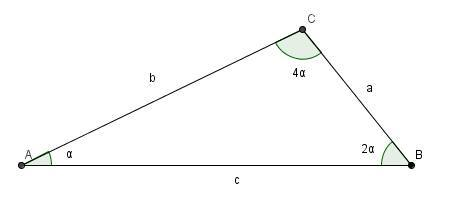# Trigonometry is Fun 3 :)

Geometry Level 2In $\triangle ABC, \angle C = 4\angle A$ and $\angle B = 2\angle A$.

What is the value of $\dfrac{b^2}{a^2} - \dfrac{c}{b}$?

×